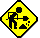AC  Equations

DESIRED
DATA

ALTERNATING CURRENT

DIRECT
CURRENT

Single-Phase

Two-Phase*
Four-Wire

Three-Phase

Amperes when
kva is shown

kva x 1000
E

kva x 1000
2 x E

kva x 1000
1.73 x E
kva x 1000
E

Amperes when
kilowatts are shown

kw x 1000
E x pf
kw x 1000
2 x E x pf
kw x 1000
1.73 x E x pf
kw x 1000
E

Amperes when
horsepower is shown

hp x 746
E x %Eff x pf
hp x 746
2 x E x %Eff x pf
hp x 746
1.73 x E x %Eff x pf
hp x 746
E x %Eff

Kilovolt-Amperes

I x E
1000
I x E x 2
1000
I x E x 1.73
1000
I x E
1000

Kilowatts

I x E x pf
1000
I x E x 2 pf
1000
I x E x 1.73 x pf
1000
I x E
1000

Horsepower

I x E x %Eff x pf
746
I x E x 2 x %Eff x pf
746
I x E x 1.73 x %Eff x pf
746
I x E x %Eff
746
 OHMS LAW  Volts (E) = Amps (I) x Ohms (R) Amps (I) = Volts (E) / Ohms (R) Ohms (R) = Volts (E) / Amps (I)   R=Ohms, E=Volts, I=Amperes POWER - AC CIRCUITS Efficiency = 746 x Output HP / Input Watts 3ø KW = Volts x Amps x PF x 1.732 / 1000 3ø Amps = 746 x HP / 1.732 x Eff. x PF 3ø Eff. = 746 x HP / 1.732 x Volts x Amps x PF 3ø PF = Input Watts / Volts x Amps x 1.732 1ø KW = Volts x Amps x PF / 1000 1ø Amps = 746 x HP / Volts x Eff. x PF 1ø Eff. = 746 x HP / Volts x Amps x PF 1ø PF = Input Watts / Volts x Amps HP (3ø) = Volts x Amps x 1.732 x Eff. x PF / 746 HP (1ø) = Volts x Amps x Eff. x PF / 746 1 KW = 1000 Watts   Eff. = Efficiency, PF = Power FactorKW = KilowattsHP = Horsepower